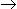# Chemical Engineering - Nuclear Power Engineering

### Exercise :: Nuclear Power Engineering - Section 1

26.

The atomic number of a radioactive element is not changed, when it emits __________ rays.

 A. α B. β C. γ D. α & β

Explanation:

No answer description available for this question. Let us discuss.

27.

Fission of U-235 on slow neutron bombardment can be represented by

 A. 92U235 + 3 γ156Ba143 + 36Kr90 B. 92U235 + 0n156Ba143 + 36Kr90 + 3 0n1 C. 92U235 + 0n156Ba143 + 36Kr90 + 3 0n1 + Q (energy) D. 92U235 + 0n192U236 + 30n1+ Q (energy)

Explanation:

No answer description available for this question. Let us discuss.

28.

A nuclear reactor can't be used for

 A. the production of radioisotopes. B. supplying intense fields or beams of neutron for scientific experiments. C. marine ship propulsion. D. none of these.

Explanation:

No answer description available for this question. Let us discuss.

29.

Which of the following is a moderating material used in nuclear reactor ?

 A. Graphite. B. Cadmium. C. Zircalloy (an alloy of zirconium and aluminium). D. Stainless steel.

Explanation:

No answer description available for this question. Let us discuss.

30.

The atomic number of an element is equal to the number of __________ present in its atom.

 A. neutrons B. electrons C. protons D. either (b) or (c)